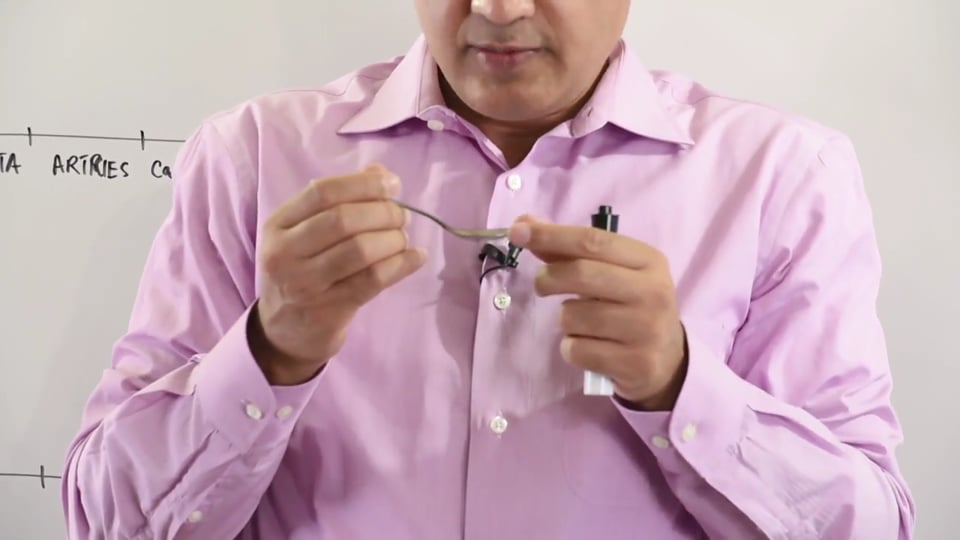# Blood Flow Velocity

{{isShortDescriptionLonger && !showFullDescription ? shortDescription.slice(0, 250) : shortDescription}}... See {{showFullDescription? 'less' : 'more'}}

#### Write A New Comment### lvhattersley@*.com

Mar 28 2019, 2:53 pm

I recall physics at school. One equation I learned was F α r4 (i.e. Flow is proportional to the 4th power of the radius) This in itself is academic. It is a proportionality, hence has a variable: the viscosity of the liquid or gas. It was taught in physics and I have never seen it expressed in a physiology class. It certainly describes the physiology of  the movement of gasses and liquids (breathing and blood flow) and, by extension, helps us understand certain pathologies: COPD anssd DVT with varicise veins, for example

This video will present: Velocity of the blood flow in aorta and smaller arteries. We will also look at the graphs that you might see in the USMLE tests. Formula to calculate the velocity.

PHYSIOLGY LECTURE # 3 STUDY NOTES

HEMODYNAMICS - BLOOD FLOW VELOCITY

The assumptions made in this lecture to understand the velocity of blood flow are as follows:

• 1 meter = 39.37 inches

• 1 millilitre = 1 cubic centimetre

• A cubic centimetre is diagrammed as a small cube which has all its lengths equal to 1 centimetre. Cardiac Output at rest: 5000mL

Let’s assume that the blood vessels are like cylinders and their cross section appear to be circular. Hence, the cross sectional area of a blood vessel is equal to the area of the circle. This can be calculated by the formula A=πr^2. Unit is cm2.

Velocity vs. Flow

Velocity is defined as the speed of blood in unit time.

Flow is the amount of blood moving per unit time.

Velocity and Flow can be integrated in the same equation as per the following formula:

​

Where:  V = velocity

Q = Flow

A = Cross sectional area of the vessel.

This shows that the velocity is inversely proportional to the cross sectional area and directly proportional to the flow of blood in the vessel. This principle is analogous to water pouring out of a water hose. Squeezing the outlet of the hose and making it narrower (decreasing the cross-sectional area) will cause the water to eject with higher than normal speed.

The cross sectional area, of any part of the vasculature is taken as the sum of all the vessels at that level and not of a single vessel individually. Hence, the aorta which is a singlevessel, has the smallest cross sectional area of 2.5cm^2. On the other hand, the sum of cross-sectional areas of all the capillaries is calculated to be 3000cm^2.

The calibre of the blood vessels changes as the aorta divides into arteries, arterioles and capillaries during the process of transporting blood to the tissues. The change in vessel calibre is met with a subsequent change in the blood velocity. The aorta, with a cross sectional area of 2.5cm^2, has blood travelling at a velocity of 20m/min. By the time the blood reaches the capillaries, the velocity of blood drops to 1.6cm/min. This is because the cross sectional area of all the capillaries when summated becomes equal to 3000cm^2, a value which is 1000 folds greater than the cross sectional area of aorta. Following calculations can be used to calculate velocity of blood flowing through the aorta and the capillaries respectively:

Velocity of the blood flow through Aorta:

➢ ​

= {ml and cm^3 can be substituted interchangeably}

=

= 2000 cm/min

= 20 m/min

= 33 cm/s

Velocity of the blood flow through the Capillaries:

➢ ​

=  {ml and cm^3 can be substituted interchangeably}

= 1.6 cm/min

= 0.016 m/min

= 0.027 cm/s

To summarize, the aorta acts as a conducting vessel as it conducts blood at high velocity to the rest of the body. The capillaries on the other hand, need to contain the blood with minimal velocity which allows for efficient exchange of gases and transport of nutrients and waste products.

In this video we will learn about:

1.Velocity of blood flow.

2.Calculation of blood flow velocity in aorta and arteries.

3.Calculattion of blood flow velocity in capillaries,veins and venules.

4.Significance of blood flow velocity.

5. Representaion of blood flow velocity through graphs.